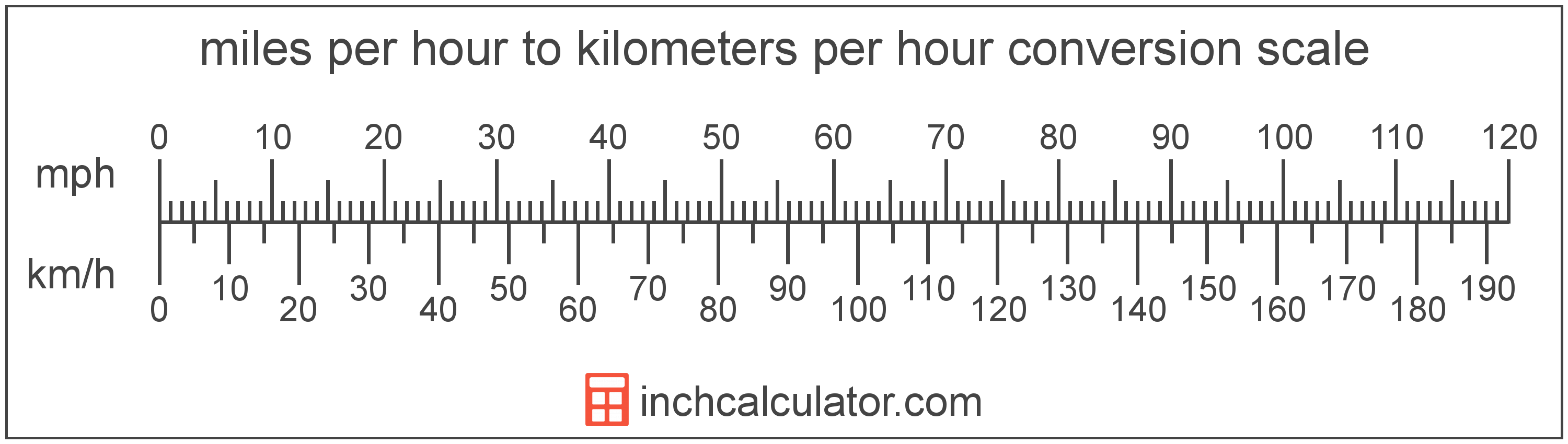# Miles per Hour to Kilometers per Hour Converter

Enter the speed in miles per hour below to get the value converted to kilometers per hour.

Results in Kilometers per Hour:1 mph = 1.609344 km/h

Do you want to convert kilometers per hour to miles per hour?

## How to Convert Miles per Hour to Kilometers per Hour

The fastest way to convert miles per hour to kilometers per hour is to use this simple formula:

km/h = mph × 1.609344

The speed in kilometers per hour is equal to the speed in miles per hour multiplied by 1.609344. Since one mile per hour is equal to 1.609344 kilometers per hour, that's the conversion ratio used in the formula.

For example, here's how to convert 5 miles per hour to kilometers per hour using the formula above.

km/h = (5 mph × 1.609344) = 8.04672 km/hMiles per hour and kilometers per hour are both units used to measure speed. Calculate speed in miles per hour or kilometers per hour using our speed calculator or keep reading to learn more about each unit of measure.

## What Are Miles per Hour?

Miles per hour are a measurement of speed expressing the distance traveled in miles in one hour.

The mile per hour is a US customary and imperial unit of speed. Miles per hour can be abbreviated as mph, and are also sometimes abbreviated as mi/h or MPH. For example, 1 mile per hour can be written as 1 mph, 1 mi/h, or 1 MPH.

Miles per hour can be expressed using the formula:
vmph = dmi / thr

The velocity in miles per hour is equal to the distance in miles divided by time in hours.

## What Are Kilometers per Hour?

Kilometers per hour are a measurement of speed expressing the distance traveled in kilometers in one hour.

The kilometer per hour, or kilometre per hour, is an SI unit of speed in the metric system. Kilometers per hour can be abbreviated as km/h, and are also sometimes abbreviated as kph. For example, 1 kilometer per hour can be written as 1 km/h or 1 kph.

In the expressions of units, the slash, or solidus (/), is used to express a change in one or more units relative to a change in one or more other units. For example, km/h is expressing a change in length or distance relative to a change in time.

Kilometers per hour can be expressed using the formula:
vkm/h = dkm / thr

The velocity in kilometers per hour is equal to the distance in kilometers divided by time in hours.

## Mile per Hour to Kilometer per Hour Conversion Table

Mile per hour measurements converted to kilometers per hour
Miles Per Hour Kilometers Per Hour
1 mph 1.61 km/h
2 mph 3.22 km/h
3 mph 4.83 km/h
4 mph 6.44 km/h
5 mph 8.05 km/h
10 mph 16.09 km/h
15 mph 24.14 km/h
20 mph 32.19 km/h
25 mph 40.23 km/h
30 mph 48.28 km/h
35 mph 56.33 km/h
40 mph 64.37 km/h
45 mph 72.42 km/h
50 mph 80.47 km/h
55 mph 88.51 km/h
60 mph 96.56 km/h
65 mph 104.61 km/h
70 mph 112.65 km/h
75 mph 120.7 km/h
80 mph 128.75 km/h
85 mph 136.79 km/h
90 mph 144.84 km/h
95 mph 152.89 km/h
100 mph 160.93 km/h

## References

1. National Institute of Standards and Technology, NIST Guide to the SI, https://www.nist.gov/pml/special-publication-811/nist-guide-si-appendix-b-conversion-factors/nist-guide-si-appendix-b8
2. Wikipedia, Miles per hour, https://en.wikipedia.org/wiki/Miles_per_hour
3. National Institute of Standards and Technology, NIST Guide to the SI, Chapter 6: Rules and Style Conventions for Printing and Using Units, https://www.nist.gov/pml/special-publication-811/nist-guide-si-chapter-6-rules-and-style-conventions-printing-and-using